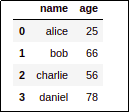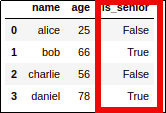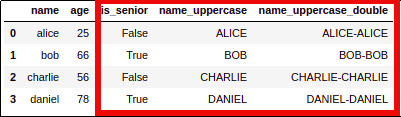Mutate for Pandas Dataframes: Examples with Assign

# Mutate for Pandas Dataframes: Examples with Assign

Last updated:

All examples on this jupyter notebook

mutate is a very popular function is R's dplyr package.

Since many people are familiar with R and would like to have similar behaviour in pandas (it's also useful for those who've never used R).

Pandas assign() function is the equivalent of mutate for pandas.

## Why use assign?

You can use it to avoid needing to define tons of intermediate dataframes in your code, especially if you're using Jupyter Notebooks or similar exploratory tools.

## Assign example

Template: df.assign(column_name = function_that_takes_a_dataframe_and_returns_a_series)

import pandas as pd

df = pd.DataFrame({
'name': ['alice','bob','charlie','daniel'],
'age': [25,66,56,78]
})

df.assign(
is_senior = lambda dataframe: dataframe['age'].map(lambda age: True if age >= 65 else False)
)BEFORE: the original dataframeAFTER: added a derived column
using the assign method

## Chain application

import pandas as pd

df = pd.DataFrame({
'name': ['alice','bob','charlie','daniel'],
'age': [25,66,56,78]
})

df.assign(
is_senior = lambda dataframe: dataframe['age'].map(lambda age: True if age >= 65 else False)
).assign(
name_uppercase = lambda dataframe: dataframe['name'].map(lambda name: name.upper()),
).assign(
name_uppercase_double = lambda dataframe: dataframe['name_uppercase'].map(lambda name: name.upper()+"-"+name.upper())
)BEFORE: original dataframeAFTER: Using .assign you can make multiple
operations that depend on the
previous ones without the need
of creating intermediate variables